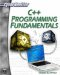# Throwing Exceptions

In addition to catching exceptions, you can simply throw them. Throwing an exception simply sends the exception back to the function that called the current function, so that the previous function can handle the exception. The following example illustrates this.

### Example 7.3

Step 1: Enter the following code in your favorite text editor.

`#include <iostream> using namespace std;   // function prototypes. float divide_number(float , float); int main() {  float dividend,divisor,answer;  try       { cout << "Please enter a number \n"; cin >> dividend; cout << "Please enter a number \n"; cin >> divisor; answer = divide_number(dividend,divisor); cout << dividend << " divided by ";      cout << divisor << " is " << answer;       }// end of try       catch(...)       {       cout << "oops, there is an error!";       }// end of catch  return 0;  } float divide_number(float num1, float num2)  {  try  {    float answer;    answer = num1/num2;    return answer;  }  catch(...)  {      throw;  }//end of catch`

Step 2: Compile the code.

You can see that exceptions are not handled in the various functions, only in the main function. Some programmers prefer to centralize their error handling in this way.C++ Programming Fundamentals (Cyberrookies)
ISBN: 1584502371
EAN: 2147483647
Year: 2005
Pages: 197
Authors: Chuck Easttom